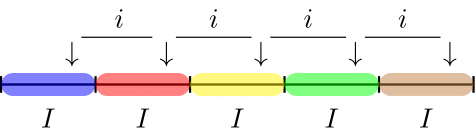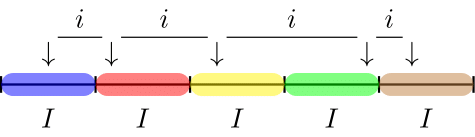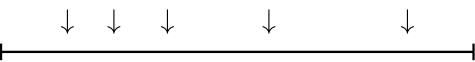# Selecting statistical audit samples

## Introduction

This vignette outlines the most commonly used sampling methodology for auditing and shows how to select a sample using these methods with the jfa package.Auditors are often required to assess balances or processes that involve a large number of items. Since they cannot inspect all of these items individually, they need to select a subset (i.e., a sample) from the total population to make a statement about a certain characteristic of the population. For this purpose, various selection methodologies are available that have become standard in an audit context.

### Sampling units

Selecting a subset from the population requires knowledge of the sampling units; physical representations of the population that needs to be audited. Generally, the auditor has to choose between two types of sampling units: individual items in the population or individual monetary units in the population. In order to perform statistical selection, the population must be divided into individual sampling units that can be assigned a probability to be included in the sample. The total collection of all sampling units which have been assigned a selection probability is called the sampling frame.

#### Items

A sampling unit for record (i.e., attributes) sampling is generally a characteristic of an item in the population. For example, suppose that you inspect a population of receipts. A possible sampling unit for record sampling can be the date of payment of the receipt. When a sampling unit (e.g., date of payment) is selected by the sampling method, the population item that corresponds to the sampled unit is included in the sample.

#### Monetary units

A sampling unit for monetary unit sampling is different than a sampling unit for record sampling in that it is an individual monetary unit within an item or transaction, like an individual dollar. For example, a single sampling unit can be the 10$$^{th}$$ dollar from a specific receipt in the population. When a sampling unit (e.g., individual dollar) is selected by the sampling method, the population item that includes the sampling unit is included in the sample.

### Sampling methods

This section discusses the four sampling methods implemented in jfa. First, for notation, let the the population $$N$$ be defined as the total set of individual sampling units $$x_i$$.

$N = \{x_1, x_2, \dots, x_N\}.$

In statistical sampling, every sampling unit $$x_i$$ in the population must receive a selection probability $$p(x_i)$$. The purpose of the sampling method is to provide a framework to assign selection probabilities to each of the sampling units, and subsequently draw sampling units from the population until a set of size $$n$$ has been created.

The next section discusses which sampling methods are available in jfa. To illustrate the outcomes for different sampling methods, we will use the BuildIt data set that can be loaded using the code below.

data(BuildIt)

#### Fixed interval sampling (Systematic sampling)

Fixed interval sampling is a method designed for yielding representative samples from monetary populations. The algorithm determines a uniform interval on the (optionally ranked) sampling units. Next, a starting point is handpicked or randomly selected in the first interval and a sampling unit is selected throughout the population at each of the uniform intervals from the starting point. For example, if the interval has a width of 10 sampling units and sampling unit number 5 is chosen as the starting point, the sampling units 5, 15, 25, etc. are selected to be included in the sample.

The number of required intervals $$I$$ can be determined by dividing the number of sampling units in the population by the required sample size:

$I = \frac{N}{n},$

in which $$n$$ is the required sample size and $$N$$ is the total number of sampling units in the population.

If the space between the selected sampling units is equal, the selection probability for each sampling unit is theoretically defined as:

$p(x) = \frac{1}{I},$

with the property that the space between selected units $$i$$ is the same as the interval $$I$$, see Figure 1. However, in practice the selection is deterministic and completely depends on the chosen starting points (using start).Figure 1: Illustration of fixed interval sampling

The fixed interval method yields a sample that allows every sampling unit in the population an equal chance of being selected. However, the fixed interval method has the property that all items in the population with a monetary value larger than the interval $$I$$ have an selection probability of one because one of these items’ sampling units are always selected from the interval. Note that, if the population is arranged randomly with respect to its deviation pattern, fixed interval sampling is equivalent to random selection.

Advantage(s): The advantage of the fixed interval sampling method is that it is often simple to understand and fast to perform. Another advantage is that, in monetary unit sampling, all items that are greater than the calculated interval will be included in the sample. In record sampling, since units can be ranked on the basis of value, there is also a guarantee that some large items will be in the sample.

Disadvantage(s): A pattern in the population can coincide with the selected interval, rendering the sample less representative. What is sometimes seen as an added complication for this method is that the sample is hard to extend after drawing the initial sample. This is due to the chance of selecting the same sampling unit. However, by removing the already selected sampling units from the population and redrawing the intervals this problem can be efficiently solved.

As an example, the code below shows how to apply the fixed interval sampling method in a record sampling and a monetary unit sampling setting. Note that, by default, the first sampling unit from each interval is selected. However, this can be changed by setting the argument start = 1 to a different value.

# Record sampling
sample <- selection(data = BuildIt, size = 100, units = "items", method = "interval", start = 1)
head(sample$sample, n = 6) ## row times ID bookValue auditValue ## 1 1 1 82884 242.61 242.61 ## 2 36 1 80125 118.58 118.58 ## 3 71 1 27566 481.44 481.44 ## 4 106 1 88261 266.66 266.66 ## 5 141 1 58999 568.60 568.60 ## 6 176 1 27801 314.65 314.65 # Monetary unit sampling sample <- selection(data = BuildIt, size = 100, units = "values", method = "interval", values = "bookValue", start = 1) head(sample$sample, n = 6)
##   row times    ID bookValue auditValue
## 1   1     1 82884    242.61     242.61
## 2  38     1 57172    329.30     329.30
## 3  73     1 90160    205.69     205.69
## 4 110     1  4756    295.96     295.96
## 5 146     1 90183    333.28     333.28
## 6 183     1 96080    449.07     449.07

#### Cell sampling

The cell sampling method divides the (optionally ranked) population into a set of intervals $$I$$ that are computed through the previously given equations. Within each interval, a sampling unit is selected by randomly drawing a number between 1 and the interval range $$I$$. This causes the space $$i$$ between the sampling units to vary.

Like in the fixed interval sampling method, the selection probability for each sampling unit is defined as:

$p(x) = \frac{1}{I}.$Figure 2: Illustration of cell sampling

The cell sampling method has the property that all items in the population with a monetary value larger than twice the interval $$I$$ have a selection probability of one.

Advantage(s): More sets of samples are possible than in fixed interval sampling, as there is no systematic interval $$i$$ to determine the selections. It is argued that the cell sampling algorithm offers a solution to the pattern problem in fixed interval sampling.

Disadvantage(s): A disadvantage of this sampling method is that not all items in the population with a monetary value larger than the interval have a selection probability of one. Besides, population items can be in two adjacent cells, thereby creating the possibility that an items is included in the sample twice.

As an example, the code below shows how to apply the cell sampling method in a record sampling and a monetary unit sampling setting. It is important to set a seed to make the results reproducible.

# Record sampling
set.seed(1)
sample <- selection(data = BuildIt, size = 100, units = "items", method = "cell")
head(sample$sample, n = 6) ## row times ID bookValue auditValue ## 1 9 1 14608 216.48 216.48 ## 2 48 1 45437 347.94 139.18 ## 3 90 1 90333 241.17 241.17 ## 4 136 1 45746 440.72 440.72 ## 5 147 1 72906 677.62 677.62 ## 6 206 1 93529 528.79 528.79 # Monetary unit sampling set.seed(1) sample <- selection(data = BuildIt, size = 100, units = "values", method = "cell", values = "bookValue") head(sample$sample, n = 6)
##   row times    ID bookValue auditValue
## 1   8     1 81460    295.20     295.20
## 2  53     1 80645    677.88     677.88
## 3  92     1 75133    355.16     355.16
## 4 142     1 68676    612.46     612.46
## 5 153     1 63777    552.83     552.83
## 6 214     1 25379   1021.07    1021.07

#### Random sampling

Random sampling is the most simple and straight-forward selection method The random sampling method provides a method that allows every sampling unit in the population an equal chance of being selected, meaning that every combination of sampling units has the same probability of being selected as every other combination of the same number of sampling units. Simply put, the algorithm draws a random selection of size $$n$$ of the sampling units. Therefore, the selection probability for each sampling unit is defined as:

$p(x) = \frac{1}{N},$

where $$N$$ is the number of units in the population. To clarify this procedure, Figure 3 provides an illustration of the random sampling method.Figure 3: Illustration of random sampling

Advantage(s): The random sampling method yields an optimal random selection, with the additional advantage that the sample can be easily extended by applying the same method again.

Disadvantages: Because the selection probabilities are equal for all sampling units there is no guarantee that items with a large monetary value in the population will be included in the sample.

As an example, the code below shows how to apply the random sampling (with our without replacement using replace) method in a record sampling and a monetary unit sampling setting. It is important to set a seed to make results reproducible.

# Record sampling
set.seed(1)
sample <- selection(data = BuildIt, size = 100, units = "items", method = "random")
head(sample$sample, n = 6) ## row times ID bookValue auditValue ## 1 1017 1 50755 618.24 618.24 ## 2 679 1 20237 669.75 669.75 ## 3 2177 1 9517 454.02 454.02 ## 4 930 1 85674 257.82 257.82 ## 5 1533 1 31051 308.53 308.53 ## 6 471 1 84375 824.66 824.66 # Monetary unit sampling set.seed(1) sample <- selection(data = BuildIt, size = 100, units = "values", method = "random", values = "bookValue") head(sample$sample, n = 6)
##    row times    ID bookValue auditValue
## 1 2174     1 90260    625.98     625.98
## 2 2928     1 68595    548.21     548.21
## 3 1627     1 98301    429.07     429.07
## 4  700     1 29683    239.26     239.26
## 5  147     1 72906    677.62     677.62
## 6 3056     1 86317    246.22     246.22

#### Modified Sieve Sampling

The fourth option for the sampling method is modified sieve sampling (Hoogduin, Hall, & Tsay, 2010). The algorithm starts by selecting a standard uniform random number $$R_i$$ between 0 and 1 for each item in the population. Next, the sieve ratio:

$S_i = \frac{Y_i}{R_i}$

is computed for each item by dividing the book value of that item by the random number. Lastly, the items in the population are sorted by their sieve ratio $$S$$ (in decreasing order) and the top $$n$$ items are selected for inspection. In contrast to the classical sieve sampling method (Rietveld, 1978), the modified sieve sampling method provides precise control over sample sizes.

As an example, the code below shows how to apply the modified sieve sampling method in a monetary unit sampling setting. It is important to set a seed to make results reproducible.

# Monetary unit sampling
set.seed(1)
sample <- selection(data = BuildIt, size = 100, units = "values", method = "sieve", values = "bookValue")
head(sample$sample, n = 6) ## row times ID bookValue auditValue ## 1 2329 1 29919 681.10 681.10 ## 2 2883 1 59402 279.29 279.29 ## 3 1949 1 56012 581.22 581.22 ## 4 3065 1 47482 621.73 621.73 ## 5 1072 1 79901 789.97 789.97 ## 6 488 1 50811 651.35 651.35 ### Ordering or randomizing the population The selection() function has additional arguments (order, decreasing, and randomize) to preprocess your population before selection. The order argument takes as input a column name in data which determines the order of the population. For example, you can order the population from lowest book value to highest book value before engaging in selection. In this case, you should use the decreasing = FALSE argument. # Ordering population from lowest 'bookValue' to highest 'bookValue' before MUS set.seed(1) sample <- selection( data = BuildIt, size = 100, units = "values", values = "bookValue", order = "bookValue", decreasing = FALSE ) head(sample$sample, n = 6)
##    row times    ID bookValue auditValue
## 1 2662     1 30568     14.47      14.47
## 2 2923     1 63567    125.21     125.21
## 3 2542     1 95807    153.56     153.56
## 4  101     1 64282    172.65     172.65
## 5  838     1 43352    188.72     188.72
## 6  302     1 94296    198.59     198.59

The randomize argument can be used to randomly shuffle the items in the population before selection.

# Randomly shuffle population items before MUS
set.seed(1)
sample <- selection(
data = BuildIt, size = 100,
units = "values", values = "bookValue", randomize = TRUE
)
head(sample\$sample, n = 6)
##    row times    ID bookValue auditValue
## 1 1017     1 50755    618.24     618.24
## 2 2159     1  3653    492.39     492.39
## 3 1639     1 39570    307.54     307.54
## 4 2698     1   225    507.18     507.18
## 5  355     1 27934    749.38     749.38
## 6 1242     1 64071    759.34     759.34